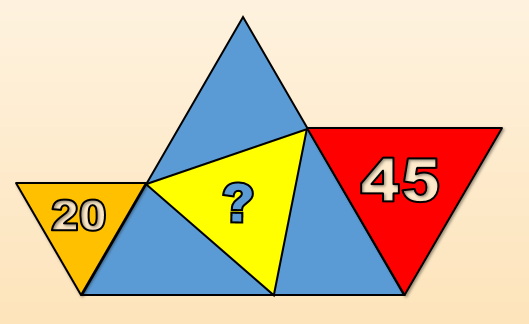# Four Equilateral triangles

Geometry Level 3There are four equilateral triangles shown in the figure. The number within each equilateral triangle represents its area.

What is the area of the yellow triangle?

×